# Top contributor algorithm

The Top Contributors algorithm identifies which combination of dimensions contributes the most to an anomaly. The figure below shows an example summary.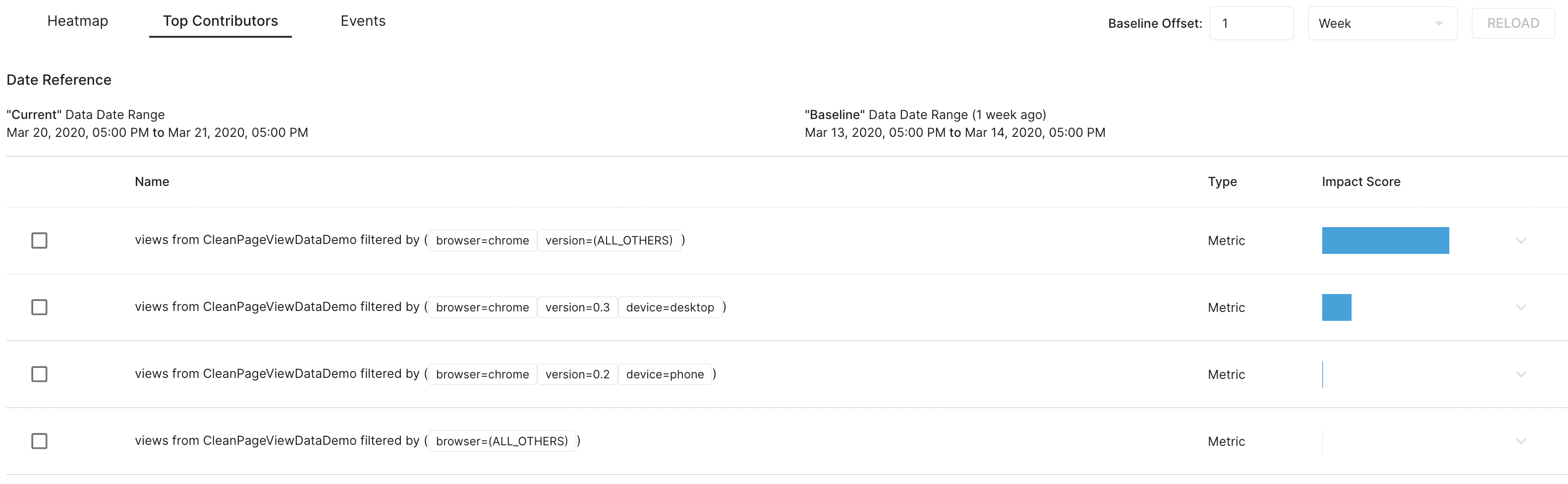By looking at the summary, we immediately know that (browser="chrome") contribute most to the overall change.
Dimension combinations are ranked by their contribution to the anomaly.

## Algorithm​

info

Below explains the algorithm used in StarTree Enterprise Edition. StarTree Community Edition uses a simpler algorithm that cannot combine multiple dimensions. Original publication from Data cube algorithm in https://engineering.linkedin.com/blog/2020/analyzing-anomalies-with-thirdeye

### Selection of nodes from dimension hierarchies​

This section describes how dimension combinations are selected and used to generate the above summary table. As an example, we will use an additive metric with three dimensions: continent, country and state. The image below shows the dimension breakdown of these dimensions, which forms a tree structure. Note that this figure has omitted some nodes. For instance, FR is a child of Europe with the children of FR omitted. In other words, every leaf of the tree is located at the third level.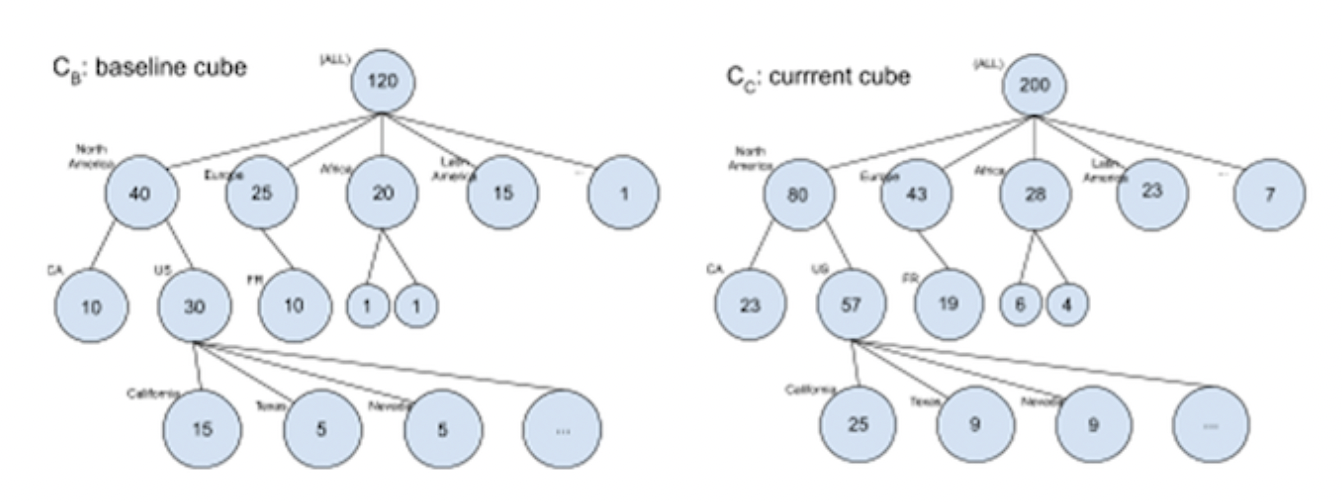In the above tree structure, the name near the circle is a dimension value for the corresponding level. When we drill down to the deeper levels of the tree, the dimension values are appended to the previous ones.

For example, the node "North America" represents the data segments whose (continent = "North America"); the node US represents the data segments whose (continent = "North America", country = "US"). The root node does not have any dimension value because it is the aggregation of all data segments.

A baseline is chosen - by default 1 week - and two trees are created: one for the anomaly period, one for the baseline. These trees are then compared to find the significant changes.

Defining change significance score

StarTree ThirdEye computes the impact of the change with three factors: change ratio, change difference, and segment contribution.

Intuitively, the change ratio measures how big the change is. Change difference measures the unexpected change compared to its parents. In other words, how much surprise the change is compared to its parent’s change. The segment contribution measures the physical impact of the node.

More formally, given the baseline and current values of a node n and its parent, the change significance score is calculated as: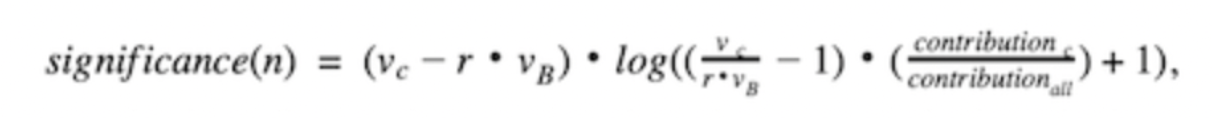where vB and vC are the baselines and current node value, respectively; r is the expected change ratio between the baseline and current from its parent node, which is defined as r = (v_parentC) / (v_parentB); contribution C is the contribution of the current node, and contribution all is the overall contribution.

Roll-up significance scores

The importance of a dimension d (e.g., country) is defined as the sum of the significance score of all its children ( e.g., (country="US"), (country="FR"), and so on). Formally, the dimension importance of d is: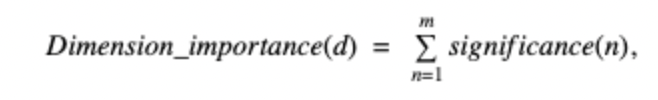where m is the children's count of dimension d. Afterward, the dimension importance is used to determine the tree structure in the figure below, i.e., the root level is the most important dimension. Finally, each parent node picks the top k (i.e., the summary size) children nodes and we merge the result of parent nodes from the bottom to the top; each merge operation keeps only the top k nodes.

Present data cube changes

Finally with the nodes selected, we can summarize the change using a table. The input of our original problem is two data cubes, in which each row is a node in the 3rd level of the tree.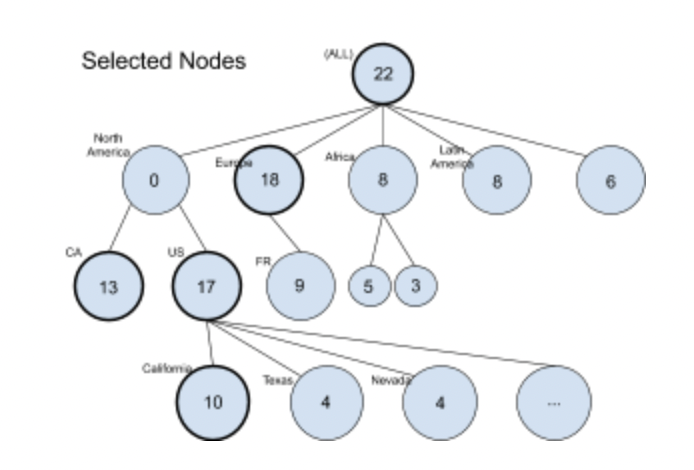Suppose that the above diagram shows the rolled-up results of our baseline and current data cubes, in which the bolded nodes are selected during the rolling-up. The following shows the table to represent the summary:

ContinentCountryState (Province)Baseline trafficCurrent traffic
OTHER(ALL)(ALL)5577
Europe(ALL)(ALL)2543
N. AmericaCA(ALL)1023
N. AmericaUSOTHER1532
N. AmericaUSCalfiornia1525

A node of the tree in the above diagram is either a data segment (leaf node) or grouped data segment (non-leaf node). In the table, the row of a leaf node contains all three dimensions. The row of a non-leaf node contains only one or two dimensions and the remaining dimensions are either (ALL) or OTHER. (ALL) indicates all its children nodes are grouped together, while OTHER means that some children are not included.

For example, the node (N. America, US, OTHER) does not include the child (N. America, US, California).